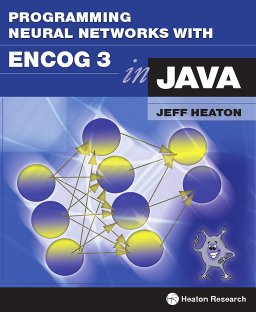[Back to books]

# Programming Neural Networks with Encog3 in Java, 2nd EditionTitle:
Programming Neural Networks with Encog3 in Java, 2nd Edition
Author:
Jeff Heaton
ISBN:
9781604390216
Pages:
226
Status:
Available
Code:
Errata:
Nothing yet.

Note: Our PDF books contain no DRM and can be printed, copied to multiple computers owned by you, and once downloaded do not require an internet connection.

You can purchase a Kindle or paperback below. Purchasing the book supports my projects and is greatly appreciated. I also allow you to download the entire book for free from this link. The free book is the complete text without any limitations; there are also ways you can support my ability to produce free content.

Note: prices above are an estimate, Amazon sets the final price. Amazon prices and currency exchange rates tend to fluxuate. Also, note that paperback and ebook may not be available in all regions.

# Description

Introduction to Neural Networks for C#, Second Edition, introduces the C# programmer to the world of Neural Networks and Artificial Intelligence. Neural network architectures, such as the feedforward, Hopfield, and self-organizing map architectures are discussed. Training techniques, such as backpropagation, genetic algorithms and simulated annealing are also introduced. Practical examples are given for each neural network. Examples include the traveling salesman problem, handwriting recognition, financial prediction, game strategy, mathematical functions, and Internet bots. All C# source code is available online for easy downloading.

• Chapter 1 provides an overview of neural networks. You will be introduced to the mathematical underpinnings of neural networks and how to calculate their values manually. You will also see how neural networks use weights and thresholds to determine their output. Matrix math plays a central role in neural network processing.

• Chapter 2 introduces matrix operations and demonstrates how to implement them in C#. The mathematical concepts of matrix operations used later in this book are discussed. Additionally, C# classes are provided which accomplish each of the required matrix operations. One of the most basic neural networks is the Hopfield neural network.

• Chapter 3 demonstrates how to use a Hopfield Neural Network. You will be shown how to construct a Hopfield neural network and how to train it to recognize patterns.

• Chapter 4 introduces the concept of machine learning. To train a neural network, the weights and thresholds are adjusted until the network produces the desired output. There are many different ways training can be accomplished. This chapter introduces the different training methods.

• Chapter 5 introduces perhaps the most common neural network architecture, the feedforward backpropagation neural network. This type of neural network is the central focus of this book. In this chapter, you will see how to construct a feedforward neural network and how to train it using backpropagation. Backpropagation may not always be the optimal training algorithm.

• Chapter 6 expands upon backpropagation by showing how to train a network using a genetic algorithm. A genetic algorithm creates a population of neural networks and only allows the best networks to ?mate? and produce offspring. Simulated annealing can also be a very effective means of training a feedforward neural network.

• Chapter 7 continues the discussion of training methods by introducing simulated annealing. Simulated annealing simulates the heating and cooling of a metal to produce an optimal solution. Neural networks may contain unnecessary neurons.

• Chapter 8 explains how to prune a neural network to its optimal size. Pruning allows unnecessary neurons to be removed from the neural network without adversely affecting the error rate of the network. The neural network will process information more quickly with fewer neurons. Prediction is another popular use for neural networks.

• Chapter 9 introduces temporal neural networks, which attempt to predict the future. Prediction networks can be applied to many different problems, such as the prediction of sunspot cycles, weather, and the financial markets.

• Chapter 10 builds upon chapter 9 by demonstrating how to apply temporal neural networks to the financial markets. The resulting neural network attempts to predict the direction of the S & P 500. Another neural network architecture is the self-organizing map (SOM). SOM?s are often used to group input into categories and are generally trained with an unsupervised training algorithm. An SOM uses a winner-takes-all strategy, in which the output is provided by the winning neuron–output is not produced by each of the neurons.

• Chapter 11 provides an introduction to SOMs and demonstrates how to use them. Handwriting recognition is a popular use for SOMs.

• Chapter 12 continues where chapter 11 leaves off, by demonstrating how to use an SOM to read handwritten characters. The neural network must be provided with a sample of the handwriting that it is to analyze. This handwriting is categorized using the 26 characters of the Latin alphabet. The neural network is then able to recognize new characters.

• Chapter 13 introduces bot programming and explains how to use a neural network to help identify data. Bots are computer programs that perform repetitive tasks. An HTTP bot is a special type of bot that uses the web much like a human uses it. The neural network is trained to recognize the specific types of data for which the bot is searching.

• The book ends with chapter 14, which discusses the future of neural networks, quantum computing, and how it applies to neural networks. The Encog neural network framework is also introduced.